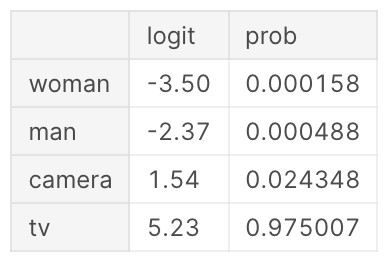## Softmax Activation FunctionThe Softmax function converts a vector of numbers into a vector of probabilities that sum to 1. It's applied to a model's outputs (or Logits) in Multi-class Classification.

It is the multi-class extension of the Sigmoid Activation Function.

The equation is:

$\sigma(\vec{z})_{i} = \frac{e^{z_i}}{\sum\limits_{j=1}^{K}e^{z_j}}$

The intuition for it is that $e^{x_i}$ is always positive and increases fast, amplifying more significant numbers. Therefore, it tends to find a single result and is less useful for problems where you are unsure if inputs will always contain a label. For that, use multiple binary columns with the Sigmoid Activation Function.

Howard et al. (2020) (pg. 223-227)

Code example:

Softmax is part of the Categorical Cross-Entropy Loss, applied before passing results to Negative Log-Likelihood function.

#### References

Jeremy Howard, Sylvain Gugger, and Soumith Chintala. Deep Learning for Coders with Fastai and PyTorch: AI Applications without a PhD. O'Reilly Media, Inc., Sebastopol, California, 2020. ISBN 978-1-4920-4552-6.